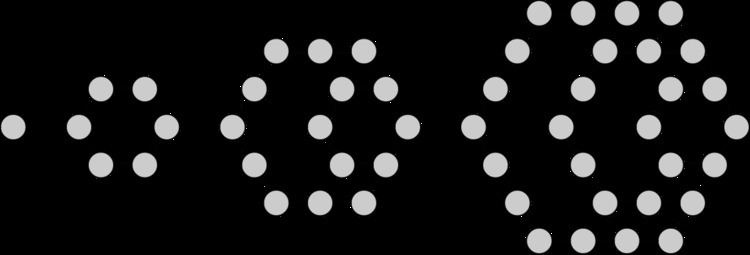# Hexagonal number

Updated onA hexagonal number is a figurate number. The nth hexagonal number hn is the number of distinct dots in a pattern of dots consisting of the outlines of regular hexagons with sides up to n dots, when the hexagons are overlaid so that they share one vertex.

## Contents

The formula for the nth hexagonal number

h n = 2 n 2 n = n ( 2 n 1 ) = 2 n × ( 2 n 1 ) 2 .

The first few hexagonal numbers (sequence A000384 in the OEIS) are:

1, 6, 15, 28, 45, 66, 91, 120, 153, 190, 231, 276, 325, 378, 435, 496, 561, 630, 703, 780, 861, 946.

Every hexagonal number is a triangular number, but only every other triangular number (the 1st, 3rd, 5th, 7th, etc.) is a hexagonal number. Like a triangular number, the digital root in base 10 of a hexagonal number can only be 1, 3, 6, or 9. The digital root pattern, repeating every nine terms, is "1 6 6 1 9 3 1 3 9".

Every even perfect number is hexagonal, given by the formula

M p 2 p 1 = M p ( M p + 1 ) / 2 = h ( M p + 1 ) / 2 = h 2 p 1 where Mp is a Mersenne prime. No odd perfect numbers are known, hence all known perfect numbers are hexagonal. For example, the 2nd hexagonal number is 2×3 = 6; the 4th is 4×7 = 28; the 16th is 16×31 = 496; and the 64th is 64×127 = 8128.

The largest number that cannot be written as a sum of at most four hexagonal numbers is 130. Adrien-Marie Legendre proved in 1830 that any integer greater than 1791 can be expressed in this way.

Hexagonal numbers can be rearranged into rectangular numbers of size n by (2n−1).

Hexagonal numbers should not be confused with centered hexagonal numbers, which model the standard packaging of Vienna sausages. To avoid ambiguity, hexagonal numbers are sometimes called "cornered hexagonal numbers".

## Test for hexagonal numbers

One can efficiently test whether a positive integer x is an hexagonal number by computing

n = 8 x + 1 + 1 4 .

If n is an integer, then x is the nth hexagonal number. If n is not an integer, then x is not hexagonal.

## Other properties

The nth number of the hexagonal sequence can also be expressed by using Sigma notation as

h n = i = 0 n 1 ( 4 i + 1 )

where the empty sum is taken to be 0.

## References

Similar Topics
Four Eyed Monsters
Tony Calder
Tanguy Pastureau
Topics